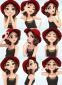# Velocity and Acceleration

2 posts / 0 new
Marie Angela RiveraVelocity and Acceleration

Can a body have a velocity without an acceleration? Can it have an acceleration with zero velocity? Give examples.

gsdeshmukh

Q.1 Can a body have a velocity without an acceleration?
ANS- Yes.
a body moving with uniform velocity doesn't have any acceleration, as acceleration is rate of change of velocity W.R.T time, for uniform velocity this rate of change is ZERO.
it means for all uniform velocities, acceleration is zero.

Q.2 Can it have an acceleration with zero velocity? Give examples.
ANS- yes.
we can have acceleration with zero velocity.
consider you thrown a ball straight up in the air. While going up, this ball has acceleration always acting in downward direction (i.e. acceleration due to gravity). after reaching maximum height this ball stops, and starts moving downward. while it is at max altitude, velocity of ball is ZERO and its acceleration is still in downward direction (i.e. acceleration due to gravity).

• Mathematics inside the configured delimiters is rendered by MathJax. The default math delimiters are $$...$$ and $...$ for displayed mathematics, and $...$ and $...$ for in-line mathematics.